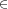## Chapter 15Solutions for third week’s assignments

Also available as PDF.

### 15.1 Section 1.4, problem 54

According to the percentages, Primestar has 16% of 12 million or 1.92 million. C-Band then has 15% or 1.8 million. Primestar has 120 thousand more subscribers.

However, the slices appear of drastically different sizes. I suspect the satellite dish is tilted “upwards” like a real dish, distorting the slices’ areas.

### 15.2 Section 2.1

#### 15.2.1 Problems 1-8

1. C
2. G
3. E
4. A
5. None of the above! They meant B, but 1 = {2}^{0} is a positive integer and a power of two. The authors meant “two raised to the power of each of the five least positive integers”. I hadn’t realized this at first, or else I would not have given this one.
6. D
7. H
8. F

#### 15.2.2 Problems 11 and 17

11. \{0,1,2,3,4\}
17. \{2,4,8,16,32,64,128,256\}

#### 15.2.3 Problems 30 and 32

30. \{x|x\text{ is an even natural number}\} is a direct translation, but \{2x|x{J}^{+}\} is shorter. Another possibility is \{x|x > 0,x\text{ is an even integer}\}.
32. One form is \{35 + 5i|iJ,0 ≤ i ≤ 12\}.

#### 15.2.4 Problems 62, 63, and 66

62. - 12\mathrel{∉}\{3,8,12,18\}.
63. 0\{- 2,0,5,9\}.
66. \{6\}\mathrel{∉}\{3,4,5,6,7\}. But note that \{6\} ⊂\{3,4,5,6,7\}.

#### 15.2.5 Problems 68, 71, 74, and 78

68. false
71. true
74. true
78. true (assuming a typical meaning for “\mathop{\mathop{…}}”)

#### 15.2.6 Problem 92

Part a. Three chocolate bars are contain a total of 660 calories. The point of this exercise is to ensure you recognize that sets are unordered, so \{r,s\} = \{s,r\} and you only include it once. The list is as follows: \{r\}, \{r,s\}, \{r,c\}, \{r,g\}, \{r,v\}, \{s,c\}, and \{s,g\}.

Part b. Five bars is 1100 calories. The list is \{r,s,v\}, \{r,s,g\}, \{r,s,c\}, \{r,c,v\}, \{r,c,g\}, and \{r,g,v\}.

### 15.3 Section 2.2

#### 15.3.1 Problems 8, 10, 12, 14

8. \{M,W,F\}⊄\{S,M,T,W,Th\}.
10. \{\text{a},\text{n},\text{d}\} ⊂\{\text{r},\text{a},\text{n},\text{d},\text{y}\}.
12. ∅⊂∅.
14. \{2,1∕3,5∕9\} ⊂ ℚ.

24. true
26. false
28. false
30. true
32. false
34. false

### 15.4 Section 2.3

1. B
2. F
3. A
4. C
5. E
6. D

#### 15.4.2 Problems 10, 17, 18, 23, 24

10. Y ∩ Z = \{b,c\}.
17. X ∪ (Y ∩ Z) = \{a,b,c,e,g\}.
18. Y ∩ (X ∪ Z) = \{a,b,c\} = Y because Y ⊂ X ∪ Z.
23. X \ Y = \{e,g\}.
24. Y \ X = \{b\}.

#### 15.4.3 Problem 31

The set consisting of all the elements of A along with those elements of C that are not in B.

#### 15.4.4 Problem 33

The set consisting of elements in A but not in C as well as elements in B but not in C. If we consider the union of A, B, and C to be the universal set, then this is the set of all elements in A complement along with all elements in B complement.

#### 15.4.5 Problems 61, 62

61. X ∪∅ = X, and the conjecture is that the union of any set with the empty set is the set itself.
62. X ∩∅ = ∅, and the conjecture is that the intersection of any set with the empty set is the empty set.

#### 15.4.6 Problems 72, 73

72. A × B = \{(3,6),(3,8),(6,6),(6,8),(9,6),(9,8),(12,6),(12,8)\}
B × A = \{(6,3),(8,3),(6,6),(8,6),(6,9),(8,9),(6,12),(8,12)\}
73. A × B = \{(d,p),(d,i),(d,g),(o,p),(o,i),(o,g),(g,p),(g,i),(g,g)\}.
B × A = \{(p,d),(i,d),(g,d),(p,o),(i,o),(g,o),(p,g),(i,g),(g,g)\}, alas, no pigdog in sight.

#### 15.4.7 Problems 117, 118, 121-124

117. A \ B = A implies that A ∩ B = ∅.
118. B \ A = A is true only when B = A = ∅.
121. If A ∪∅ = ∅, then A = ∅.
122. A ∩∅ = ∅ for any set A.
123. If A ∩∅ = A, then A = ∅.
124. A ∪∅ = A for all sets A.